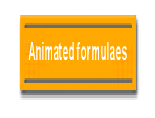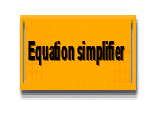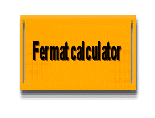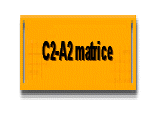I#### Vers la version française

n this page, you will find animations and graphic explanations for the schoolchildren, small drawing sometimes costing more than long speech

Hey , draw me maths....

# Summing fractionsMany pupils miss understand the common denominator in the addition (or subtraction) of fraction.

I conceived this small graphic demonstration to defuse him with some pencil strokes.

Summing 1/2 and 2 /3

For the identical rendrent (in the same denominator) I cut the first one  by the number of strips of the second

The first by 3 and the second in 2

We count now 6 identical rectangles in every square: THE COMMON DENOMINATOR, the Divisor

We consider now numbers of squares yellow total:

3 in the first one, 4 in the second is a total of 7

Total is 7/6

(1*3)/(2*3) + (2*2)/(3*2)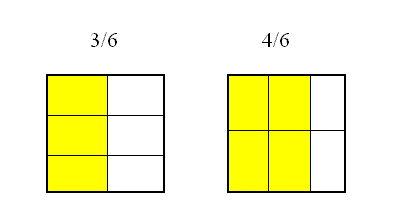# Pythagore : animated démontration of b²=c² - a² with topologic squares

images/anims/carre_pair.swf

This link allow a full screen display

If a² + b² = c²  then b² = c² - a²

On this simple example (named even topology) a square b ,dividable by 4, is cut in 4 slices of 4 units

Surface equal 4x4 forming b² (b=4 , = 16)

Those 4 slices form 2 new squares:

1 / The small white square (in the center of the yellow crown) form the square has measuring 3 units and a surface of 3 x 3: a²

2 / The largest square (the outer edge of the yellow courone) shape square c measuring 5 units and an area of 5 x 5: c²

C squared is the sum of the yellow courrone: b², and the white square to the a² Center

You can reproduce this demo with a square of paper...

Manufacture of an infinite number of rectangles triangles with the "Fermat calculator"

# Animated Remarquables identities

## (a+b)² = a²+2ab+b²

http://schemath.com/images/anims/a_plus_b_carre1.swf

This link allow a full screen display

## (a-b)² = a²-2ab+b²

http://schemath.com/images/anims/a_moins_b_carre1.swf

This link allow a full screen display

## a² - b² = (a+b)(a-b)

http://schemath.com/images/anims/a2_b2_apb_amb.swf

This link allow a full screen display

# Tools

Download Equation simplifier for learning expanded formulaes